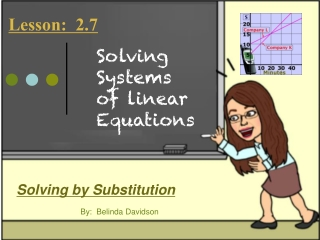DownloadDownload PresentationSolving Systems of linear Equations

# Solving Systems of linear Equations

Download Presentation## Solving Systems of linear Equations

- - - - - - - - - - - - - - - - - - - - - - - - - - - E N D - - - - - - - - - - - - - - - - - - - - - - - - - - -
##### Presentation Transcript

1. Lesson: 2.7 Solving Systems of linear Equations Solving by Substitution By: Belinda Davidson

2. Quick Review • Solve the equation for x: 2y + x =12 • x = -2y + 12 • How does the graph of a system look when there is “no solution”? • The lines are parallel and never intersect • (inconsistant) • How does the graph of a system look when there are “infinitely many solutions”? • The two lines are the same line. • (dependent)

3. Solving by Substitution • Pick an equation (the one that’s easiest to get one of the variables by itself) and solve for a variable • Substitute into the OTHER equation • Solve for the first variable • Substitute and solve for the second variable (doesn’t matter which equation) • Check your solution (YES, you need to check your answer!)

4. Pick Equation • Substitute • Solve • Substitute and Solve • Check Solving by Substitution Solve the linear system -x + y = 4 -8x + 2y = 2 -x + y = 4 +x +x y = x + 4 Check ( 1, 5) -x + y = 4 -1 + 5 = 4 4 = 4  -8x + 2y = 2 -8(1) + 2(5) = 2 -8 + 10 = 2 2 = 2  -8x + 2(x + 4) = 2 -8x + 2x + 8 = 2 -6x + 8 = 2 -6x = -6 x = 1 -x + y = 4 -(1) + y = 4 y = 5 (1, 5)

5. You Try • Pick Equation • Substitute • Solve • Substitute and Solve • Check Solve the linear system 1. y = 2x – 4 x = y + 3 2. –m + n = 1 2m + n = -2 3. 2c + 2d = 3 c – 4d = -1 ( 1, -2) (-1, 0) (1, ½)

6. Application Your school must transport 193 people to a competition. There are eight drivers available and two types of vehicles. The school buses seat 51 people each, and the minivans seat 8 people each. How many buses and minivans will be needed? b = number of school buses m = number of mini vans b + m = 8 51b + 8m = 193 (3, 5)

7. No solution/Infinite solutions Infinite Solution No Solutions No Solutions Infinite Solution • y = 3x + 2 6x – 2y = -4 2. y = 2x + 6 4x – 2y = 8 3. 2x + y = -1 -6x – 3y = -15 4. 15x – 5y = -20 -3x + y = 4

8. Concept: Application Ex 1 Find the value of two numbers whose sum is 12 and difference is 4. { 1. Write an equation for the sum x + y = 12 2. Write an equation for the difference x – y = 4 3. Solve the system. x + y = 12 –x –x y = –x + 12 x – y = 4 x – (–x + 12) = 4

9. Concept: Application Ex 1 { 3. Solve the system. x + y = 12 x – y = 4 x + x – 12 = 4 2x – 12 = 4 8 – y = 4 + 12 + 12 –8 –8 2x = 16 __ __ 2 2 –y = –4 __ __ -1 -1 x = 8 y = 4 Solution: (8, 4)

10. Wrap Up Substitution Method • Pick an equation • Substitute • Solve • Substitute and solve • Check No Solution (Inconsistant) x and y cancels and two sides are different Infinitely Many Solutions (Dependent) x and y cancels and both sides are equal

11. HomworkIXL A2:E.6